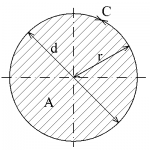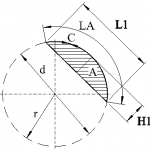# Circle calculations

## Circle defintion

A circle is a set of points in a plane whose distance from a fixed point (the center of a circle) is less than or equal to a fixed positive number (called a radius).Circumference and area of a circleCircumference and area of a circle segment